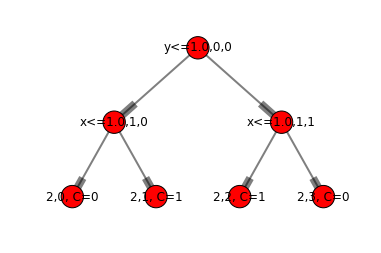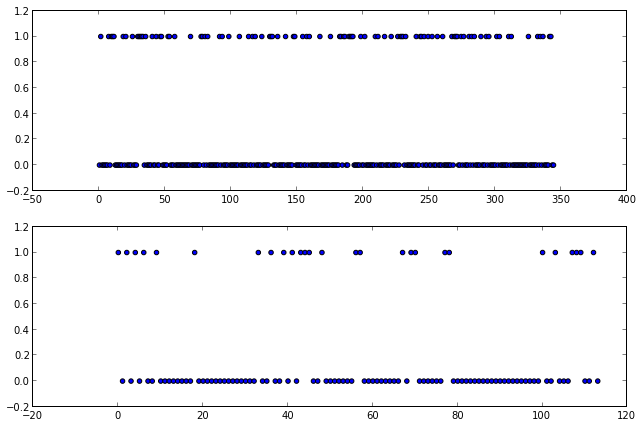# Decision Trees

## Introduction

Decision trees divide the input space into some number of cuboid regions. Every input within a given region is assigned the same output. A decision tree model can be represented by a binary tree where

• Each tree node tests one attribute from the input vector $X$

• Each tree branch selects one value (or range) for the given attribute

• Each leaf node predicts an output, Y

Decision tree models cab be applied to both regression and classification problems, however the focus here will be on classification trees. Note also, that decision trees can represent any boolean function. The tree will have $2^N$ leaf nodes where $N$ is the number of boolean variables, however there are $2^{2^N}$ possible trees given $N$ variables. For other decision trees (i.e. for non-Boolean variables) the optimal number of leaves is unknown and the input vector selection attribute may be repeated at multiple nodes.

The algorithms presented here are the so-called CART (classification and regression trees) methods. Another popular method, is the C5.0 (formally ID3) method presented by Quinlan. The resulting tree models will be binary decision trees (i.e. there are exactly 2 edges from each node except for leaf nodes)

## Classification Trees

Assume our training data consists of $N$ input vectors each with $p$ elements and an associateed single output from a set of $K$ classes, i.e. each input is of the form $X_i = \\{x_{i1}, x_{i2}, \ldots , x_{ip}\\}$ and with an associated output $y_i \in \\{c_1, c_2, \ldots, c_K\\}$ where $i \in \\{1 \ldots N\\}$. Assume the decision tree model has $M$ leaf nodes, i.e. the input space is divided into $M$ regions, denoted $R_m$. Every input in the region $R_m$ will be assigned to class $k(m)$ where

$k(m) = \max_k p^e_{mk} = \max_k\frac{1}{N_m} \sum_{x_i \in R_m} I(y_i = k)$

where $I$ returns 0 if $y_i=k$ and 0 otherwise, $p^e$ is the estimate of the probability of the observation being in class $k$ given the training data, and

$N_m = num\\{x_i \in R_m\\}$

is the number of inputs from the training set in region $R_m$. NOTE: I would prefer to use $\hat{p}$ instead of $p^e$ but there appears to be a bug in IPython Notebook.

### Growing The Tree

The standard approach to creating a decision tree model is to use a greedy algorithm. The tree is started with a single root node that splits the data into two regions based on the selection of a single input feature, $j$, and an associated splitting value, $s$. That is, $j$ is the choice of feature and $s$ is the value of that feature used to split the training data. From there new nodes are added by successively repeating this process for the training data subregions resulting from the previous split. This process is continued until some stopping criteria is reached. From there, the tree pruning is applied as a means to prevent overfitting the data.

#### Split Criteria

The first task is to define how to select the split variable, $j$, and value $s$. This is done by minimizing an impurity measure, $Q_m(T)$, summed over the two nodes resulting from the split. Some of the more impurity measures for classification problems are

Misclassification error: $\frac{1}{N_m} \sum_{i\in R_m} I \left( y_i \ne k(m) \right) = 1 - p^e_{mk}(m)$

Gini Index: $\sum_{k \ne k'} p^e_{mk} p^e_{mk'} = \sum_{k=1}^{K} p^e_{mk} \left(1 - p^e_{mk} \right)$

Cross-entropy: $- \sum_{k=1}^K p^e_{mk} \log p^e_{mk}$

Given a choice of $Q_m$, it is a straightforward matter of iterating over each feature, $j$, and split value $s$ to determine the optimal choice. Note that even if a feature is continuous, implying an infinite space of possible split values, the training data set, from which $s$ must be selected, is finite.

The stopping criteria is generally chosen as some number, $D$, of training values associated with the leaf nodes. That is, if any region $R_m$ contains greater than $D$ inputs from the training data, continue the splitting process.

TODO

#### Pruning

Given a starting tree, $T_0$, pruning is done by minimizing the cost complexity function

$C_{\lambda}(T) = \sum_{m=1}^{|T|} N_m Q_m(T) + \lambda |T|$

where $T \subset T_0$, $|T|$ is the number of leaf nodes in $T$, and $\lambda$ is a tuning parameter.

Reduced-Error Pruning 1. Split data inot training and validation set 2. Create tree that classifies training set data Prune until further pruning is harmful 1. Evaluate impact on validation data of pruning each possible node 2. Greedily remove the node that most improves the validation accuracy

Rule Post-Pruning 1. Convert tree to equivalent set of rules 2. Prune each rule independently of others 3. Sort final rules into desired sequence for use

## Example 1

Uses artificially generated data in 4 regions.

In :
sys.path.append('pysrc')
import decision_trees as dt
import networkx as nx
import numpy as np

inputs = np.zeros((16, 2))
outputs = []
row = 0
for x in range(4):
for y in range(4):
inputs[row] = x
inputs[row] = y
row += 1

for row in inputs:
if (row > 1 and row < 2) or (row < 2 and row > 1):outputs.append(1)
else: outputs.append(0)

clazz = [0,1]
meta = ['x','y']
tree = dt.build_tree(inputs, outputs, clazz, meta)
dt.draw_tree(tree)
data = np.zeros((16,4))
for r in range(16):
data[r] = r
data[r] = inputs[r]
data[r] = inputs[r]
data[r] = outputs[r]
#print data## Example 2

Uses data provided from the "The Elements of Statistical Learning" website on South African heart disease here.

In :
sys.path.append('pysrc')
import decision_trees as dt
import numpy as np
import networkx as nx
from matplotlib import pyplot as plt

p = 'data/SAheart.data'
f = open(p,'r')
train_cnt = int(0.75 * len(all_lines))
lines = all_lines[0:train_cnt]
col_cnt = len(lines.split(','))
row_cnt = len(lines)
outputs = [int(line.split(',')[col_cnt-1]) for line in lines]
inputs = np.zeros((row_cnt, col_cnt-1))
for row in range(row_cnt):
line = lines[row].split(',')[0:col_cnt-1]
inputs[row] = [float(v) for v in line]
clazz = [0,1]

tree = dt.build_tree(inputs, outputs, clazz, meta=['sbp','tob','ldl','adip','famhist','typea','obes','alc','age'], max_rm=5)
#dt.draw_tree(tree)

#compare the tree precition to training values, since we haven't pruned this should be very accurate
diff = []
for row in range(train_cnt):
p = dt.decide(tree, inputs[row])
if p == outputs[row]: diff.append(0)
else: diff.append(1)

misses = sum(diff)
print "In the training data, there were {0} miss classifications for {1} inputs, a rate of {2}%".format(misses, train_cnt, 100*misses/float(train_cnt))
x = range(train_cnt)
f, axarr = plt.subplots(2,1)

#plot training comparison
ax1 = axarr
ax1.scatter(x,diff)

#compare the tree prediction to actual values not used in training set
test_lines = all_lines[train_cnt+1:len(all_lines)-1]
actual_out = [int(line.split(',')[col_cnt-1]) for line in test_lines]
row_cnt = len(test_lines)
test_in = np.zeros((row_cnt, col_cnt-1))
for row in range(row_cnt):
line = test_lines[row].split(',')[0:col_cnt-1]
test_in[row] = [float(v) for v in line]

diff = []
for row in range(len(test_in)):
p = dt.decide(tree, test_in[row])
if p == actual_out[row]: diff.append(0)
else: diff.append(1)
misses = sum(diff)
print "In the hold out data, there were {0} miss classifications for {1} inputs, a rate of {2}%".format(misses, len(test_in), 100*misses/float(len(test_in)))

x = range(len(diff))
ax2 = axarr
ax2.scatter(x,diff)

In the training data, there were 95 miss classifications for 345 inputs, a rate of 27.5362318841%
In the hold out data, there were 27 miss classifications for 114 inputs, a rate of 23.6842105263%

Out:
<matplotlib.collections.PathCollection at 0x1050acfd0>In :